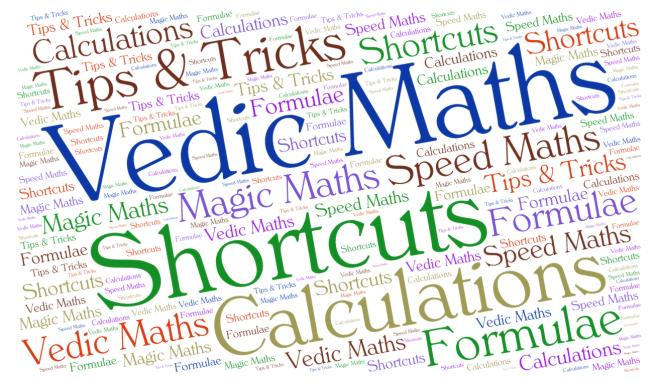` Vedic Maths Course for the age 7-11 Std. | Aloha Speed Maths Course

# Speed Maths (Vedic Maths) - 6th Std. Onwards

Multiply numbers like 999988899 x 999998889 take only 20 seconds to solve by Aloha kids.

Speed Maths (Vedic Maths) course is based on Indian mathematics of Vedic period. Aloha provides complete training and guidance to the kids for their better performance.## Why Vedic Maths Course is so important?

Vedic Maths is a well-known technique for calculation, the simplicity of Vedic Mathematics is that calculations can be carried out mentally (though the methods can also be written down). There are many advantages in using a flexible, mental system. Kids can invent their own methods, they are not limited to the one 'correct' method. This leads to more creative, interested and intelligent children.

Aloha centres provide classroom training for Speed Maths (Vedic Maths) throughout Gujarat.

## Speed Maths (Vedic Maths) makes math more interesting and fun

By helping child perform some impossible calculations at lightning speed.

By a right mix of fun, memory, techniques, memory recall and application of formulae which also makes your child a super performer.

## Benefits of enrolling you kid in Speed Maths (Vedic Maths).

• Square roots, multiplication, division, the solution to equations and much tougher calculations can be arrived at without using a tool.
• Calculations are carried out mentally through vedic maths course.
• Saves time during Examination.
• Cross checking answers is easy
• SPEED MATHS (vedic maths) is a zero error technique.
• Vedic Mathematics technique can be applied even for numbers beyond 10 digits.
• Calculate 10 times faster than the usual way.
• In multiplication only one step to arrive at the right answer.
• Short procedures applied for calculation, makes the child get more interest in the subject.
• Due to the short and simple procedures, rechecking is very easy.

## Why vedic maths training?

Vedic maths course helps to improve calculation speed. Learning all the Vedic math sutra by heart helps to solve any kind of complex calculation in seconds, in addition to that it improves accuracy and concentration power of the mind. Vedic maths course creates a love for math to a child. Vedic math is an old age technique of calculation, and as it is always said that old is gold so is the Vedic Math Course.

## Supplementary Features:

In addition to Vedic Maths classes, we provide supplementary features to make your kid's education more interesting.

Puzzles: 12 puzzles per level to develop the critical analysing skills.

Games: 12 games per level to apply mathematical concepts in an interesting manner.

Activities: 12 activities per level to enhance the learning capacity of students

#### Course Structure

Levels: 3 levels

Each of 4 month duration

Duration:
1 class per twice in a week of 2 hour

Performance evaluation is carried out at the end of each level.

Certifications:
Level 1
Level 2
Level 3

To Top ↑